Function Repository Resource:

# HydrogenWavefunction

The position-space wavefunction of the hydrogen atom

Contributed by: Matt Kafker
 ResourceFunction["HydrogenWavefunction"][{n,l,m},a,{r,θ,ϕ}] gives the wavefunction for the hydrogen atom with quantum numbers (n,l,m) and Bohr radius a as a function of the spherical coordinates r,θ and ϕ. ResourceFunction["HydrogenWavefunction"][{n,l,m},a,{r,θ,ϕ},Z] gives the hydrogen-like wavefunction with nuclear charge Z.

## Examples

### Basic Examples (2)

The hydrogen ground state wavefunction:

 In:=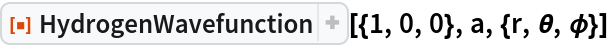Out=The squared magnitude of the wavefunction gives the probability distribution for finding the electron:

 In:=Out=### Scope (3)

Plot radial dependence of a few wavefunctions:

 In:=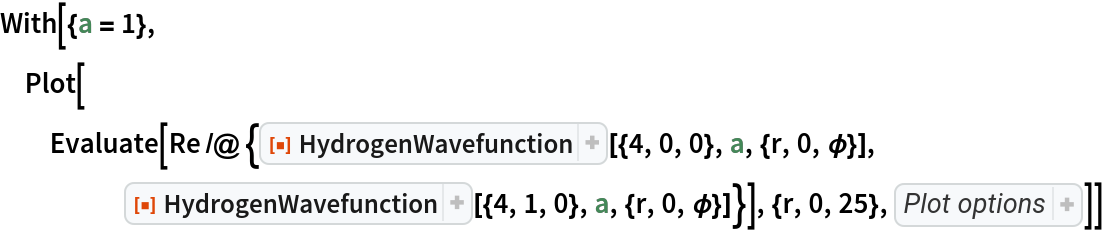Out=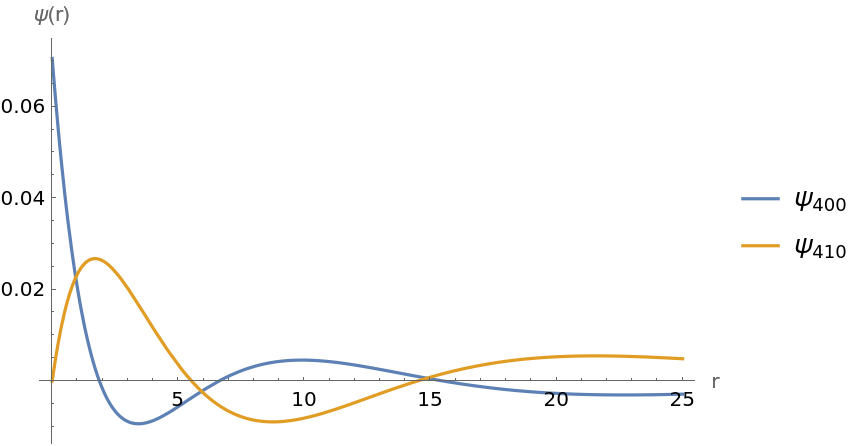Plot the polar dependence of one wavefunction at various radii:

 In:=Out=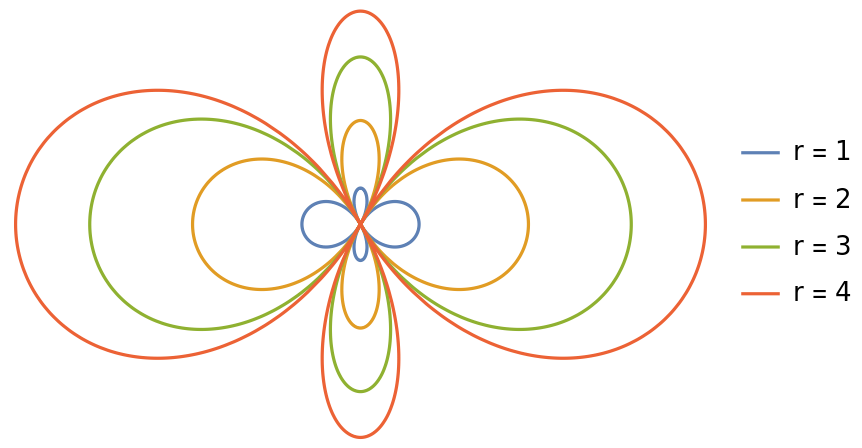Plot the electron probability density for various wavefunctions:

 In:=Out=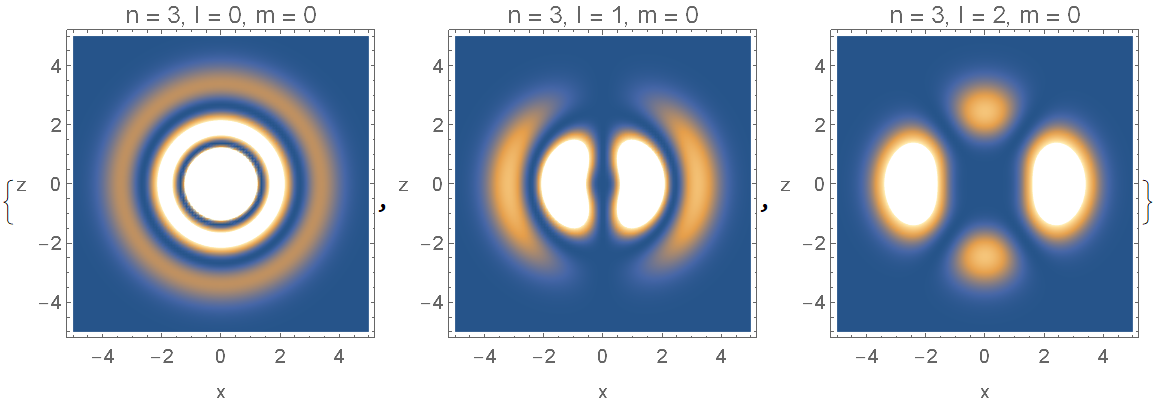### Properties and Relations (4)

Verify the orthogonality property of HydrogenWavefunction:

 In:=Out=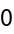Verify the normalization property of HydrogenWavefunction:

 In:=Out=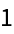Verify that HydrogenWavefunction satisfies the time-independent Schrödinger equation:

 In:=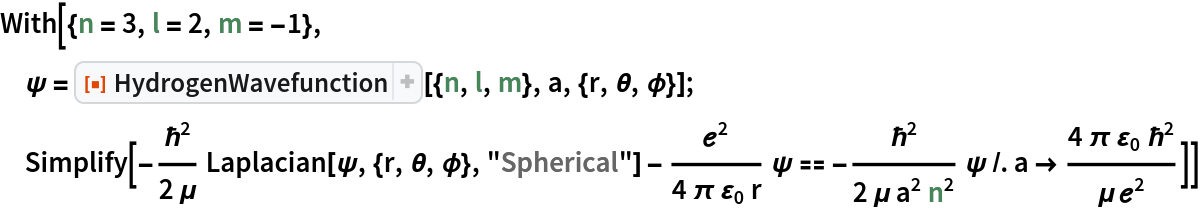Out=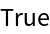Show a change of nuclear charge:

 In:=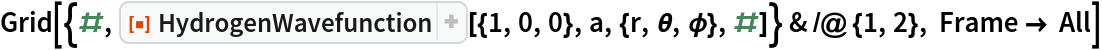Out=### Neat Examples (1)

Plot hydrogen orbital densities:

 In:=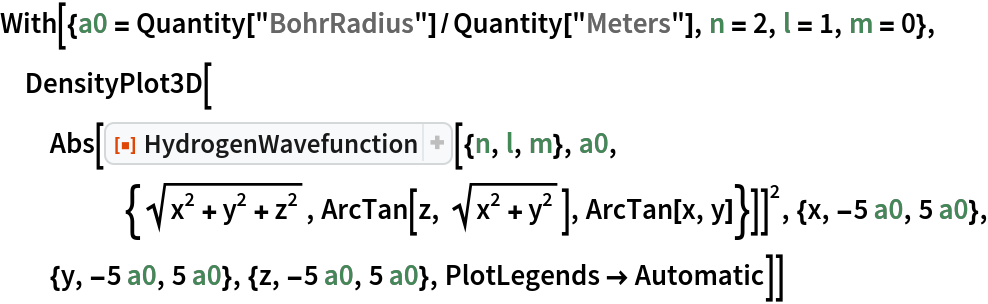Out=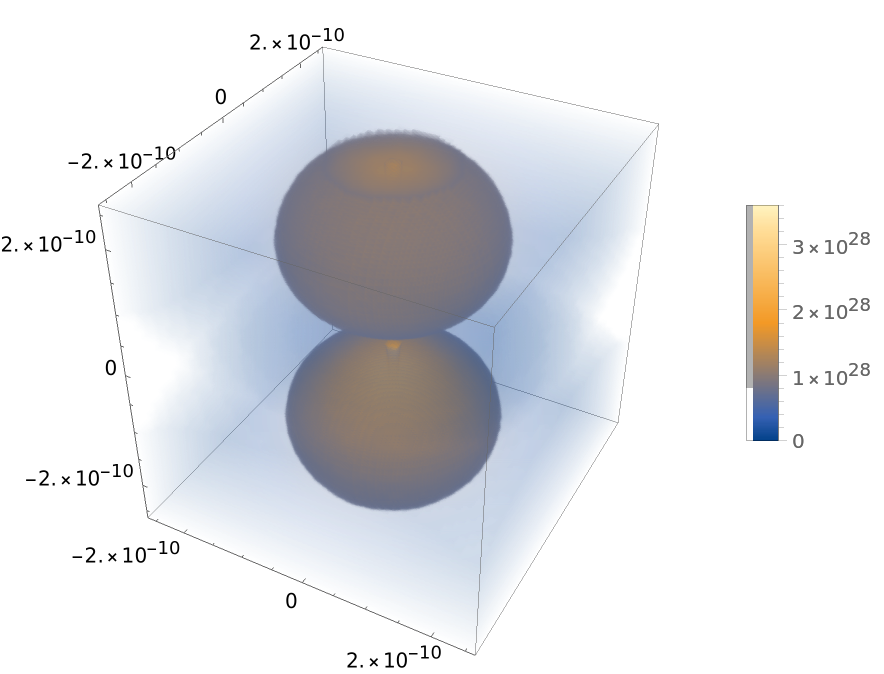## Publisher

Wolfram Summer School

## Version History

• 1.0.0 – 07 June 2021